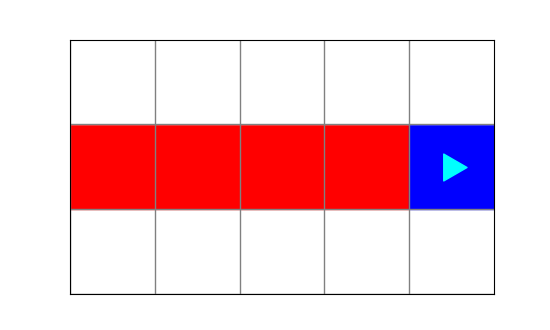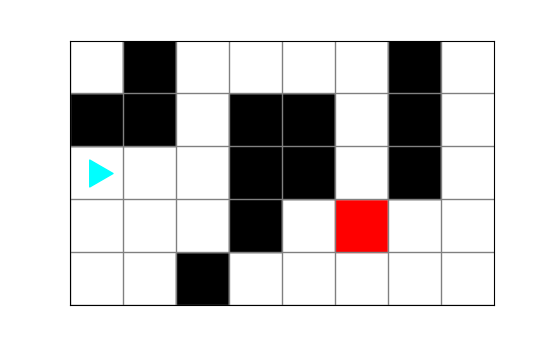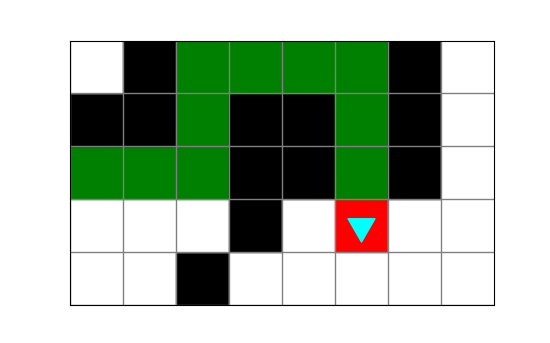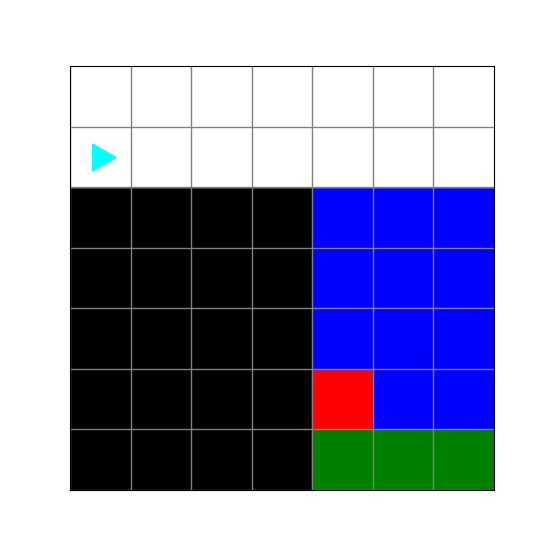Computer Science

# Lab 1c — While

## Preparation

### 5 minutes

Download the zip file for this lab, located above. This zip file has worlds that you will use for Bit. Extract the files and put them in your `cs110` directory in a folder called `lab1c`.

## Exercise 1

### 5 minutes

(a) Can you figure out what this code does?

On a piece of scratch paper, draw out what you think this code will do:

``````from byubit import Bit

def green_line(bit):
while bit.front_clear():
bit.move()
bit.paint('green')

def blue_line(bit):
while bit.front_clear():
bit.paint('blue')
bit.move()

@Bit.empty_world(5, 3)
def go(bit):
green_line(bit)
bit.left()
blue_line(bit)

if __name__ == '__main__':
go(Bit.new_bit)
``````

(b) Run the code

Inside of `lab1c`, create a file called `exercise1.py`. Copy and paste the above code into this file.

## Exercise 2

### 5 minutes

(a) Can you figure out what this code does?

On a piece of scratch paper, draw out what you think this code will do:

``````from byubit import Bit

@Bit.worlds('red-spot')
def go(bit):
while not bit.is_red():
bit.move()

bit.left()
bit.move()
bit.left()

while bit.front_clear():
bit.move()
bit.paint('blue')

if __name__ == '__main__':
go(Bit.new_bit)
``````

This code uses the following world:(b) Run the code

Inside of `lab1c`, create a file called `exercise2.py`. Copy and paste the above code into this file.

Be sure that the `worlds` directory is also in your `lab1c` folder. You should have gotten this from the downloaded zip file.

## Exercise 3

### 5 minutes

Inside of `lab1c`, create a file called `exercise3.py`. Copy and paste the following code into this file.

``````from byubit import Bit

@Bit.empty_world(5, 3)
def go(bit):
pass

if __name__ == '__main__':
go(Bit.new_bit)
``````

Using this code, create this image:Now delete that code and create this image:## Exercise 4

### 10 minutes

Inside of `lab1c`, create a file called `exercise4.py`. Copy and paste the following code into this file.

``````from byubit import Bit

def move_while_clear(bit):
""" Moves while front is clear. Paints green as it goes. """
pass

def move_while_not_red(bit):
""" Moves while current square is not red. Paints green as it goes. Does not paint the red square green. """
pass

@Bit.worlds('maze')
def solve_maze(bit):
pass

if __name__ == "__main__":
solve_maze(Bit.new_bit)``````

This code uses the following world:Your goal is to have Bit navigate the maze and make it to the red square:While Bit moves, it paints a green path.

To help you with the decomposition, we have given you two functions that you can complete. The `solve_maze()` function should call these two functions, with some glue code.

### Note

It is often helpful to break problems into smaller pieces. Try this:

a) Fill out `move_while_clear()` first, and call it once from inside `solve_maze()`. You should be able to paint green while running into the wall.

b) Now call `move_while_clear()` multiple times inside of `solve_maze()` with some glue code. Get Bit to the last turn.

c) Finally, fill out `move_while_not_red()` and call it inside of `solve_maze()`. This should finish the problem.

## Exercise 5

### 10 minutes

Inside of `lab1c`, create a file called `exercise5.py`. Copy and paste the following code into this file.

``````from byubit import Bit

@Bit.worlds('dive-for-treasure', 'dive-for-deep-treasure')
def dive(bit):
pass

if __name__ == "__main__":
dive(Bit.new_bit)
``````

Bit starts in a world that looks like this:This represents a cliff, the ocean, and a treasure (red square) at the bottom of the ocean.

First get Bit to the ocean, then dive to the red treasure and take it (leaving blue ocean behind). Your ending world should look like this:Be sure to write several helper functions instead of putting all of your code in `dive()`. The `dive()` function should just call your helper functions, with any glue code that is needed.

After you solve this problem, you will notice there is a second world, called `dive-for-deep-treasure`. You should click the tab for the second world and be sure your solution works for that world too.

## Extras

If there is extra time in the lab section, the TA may help you answer these questions:

• When a while loop finishes, what line of code is run next?
• What happens when you put one while loop inside of another while loop?
• Using nested while loops can get complicated. How can functions help with that?
• How are nested loops different than sequential loops?
``````# Sequential:
while bit.front_clear():
bit.move()
bit.right()
while not bit.is_red():
bit.move()``````